Livio/ November 3, 2019/ Python/ 0 comments

Collaborative Filtering is a technique used in recommender systems to make predictions about the interests of a user by collecting preferences for many users. The underlying assumption of the collaborative filtering approach is that if a person A has the same opinion as a person B on an issue, A is more likely to have B’s opinion on a different issue than that of a randomly chosen person. For instance, given the scenario below where users are rating movies from 1 to 5:collaborative filtering can learn parameters for each user and movie and help us predict the rating User 1 would give to Movie 2 and Movie 5 and User 2 would give to movie 4. This could then in turn be used to recommend movies to a specific user.

Problem Specification

Our task will be to have the algorithm learn the the parameters for a user J: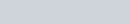and for a movie i: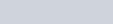in order to have the rating that user J gives to a movie i equal to:Note: the number of features, m in the case above, is a hyperparameter which is chosen during validation. To make it easier to follow, I will choose a value of 2 going forward.

Going back to example where we had 5 movies and 3 users, the prediction of all the ratings is therefore:and the actual ratings are:Cost Function

The cost function will have to be calculated only for those combinations of movie/user where a rating is available. For this purpose, we define a matrix R made up of 0’s and 1’s. A 0 indicates a missing rating, a 1 indicates a provided rating. Continuing with the example above, R is equal to:The cost function to minimize is the following: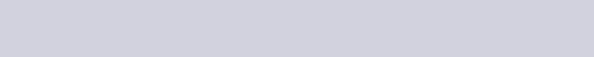where lambda is the regularization hyperparameter

In order to minimize the cost function, we need to calculate the partial derivatives for each of the parameters. After some simple calculus, it is possible to show that the derivatives with respect to the matrix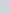is equal to: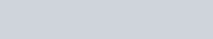and the one with respect to the matrix X is equal to: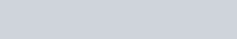when calculating the derivatives, we need to discard the combinations of users/movies with no rating provided once again.

Let’s start coding

Now that we have the math out of the way, let’s start writing the class.

__init__ method:

the init method will accept our 2 hyperparameters plus the maximum number of iteration when finding the parameters that minimize the cost function:

_initialize_thetas method:

this method initializes the thetas parameters with random values between 0 and 1:

_reshape_thetas method:

This method is used to reshape the 1 dimensional parameters vectors into their respective matrices

This method is used to calculate the partial derivatives for each of our parameters:

_cost method:

This method calculates the value of the cost function:

fit method:

predict method:

Used to predict the rating a certain user would give to a certain item

recommended_items method:

Used to return the indexes (sorted in descending order) of the top items a certain user would rate the best

compute_similarities method:

This method returns the cosine similarity matrix if either the users or the items. Each user or item is represented by a features vector of length ‘num_features‘ .

The cosine similarity is a measure of the cosine of the angle between two non zero vectors. It goes from -1 to 1. It is 1 when two vectors point in the same direction and -1 then they point in opposite directions.

The results of this method can be used to find out the users most similar to a certain user or the items most similar to a certain item

The whole class

An example of how to use the class: